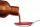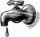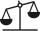# 3 children

3 children had to divide 4 pounds is candy. How much candy did each child get?

Result

x =  1.333

#### Solution:Leave us a comment of example and its solution (i.e. if it is still somewhat unclear...):

Showing 0 comments:Be the first to comment!#### To solve this example are needed these knowledge from mathematics:

Need help calculate sum, simplify or multiply fractions? Try our fraction calculator.

## Next similar examples:

1. ChocolateChildren break chocolate first to third and then every part of another half. What kind got each child? Draw a picture. What part would have received if each piece have halved?
2. Pounds3 pounds subtract 1/3 of a pound.
3. DosesA child is to receive a dose of 0.5 teaspoon of cough medicine every 12 hours. if the bottle contains 60 doses, how many days will the medicine last?
4. The tapFor one day flows 148 l of water out of the tap. How much water will flow out for 3/4 day?
5. BrickIsosceles scale has on one side all brick and second weight 1 kg and 1/4 of brick. The balance is in equilibrium. What is the weight of a brick?
6. Fraction and a decimalWrite as a fraction and a decimal. One and two plus three and five hundredths
7. Lengths of the poolMiguel swam 6 lengths of the pool. Mat swam 3 times as far as Miguel. Lionel swam 1/3 as far as Miguel. How many lengths did mat swim?
8. CakesOn the bowl were a few cakes. Jane ate one-third of them, Dana ate a quarter of those cakes that remained. a) What part (of the original number of cakes) Dana ate? b) At least how many cakes could be (initially) on thebowl?
9. Fraction to decimalWrite the fraction 3/22 as a decimal.
10. Mixed2improperWrite the mixed number as an improper fraction. 166 2/3
11. ZdeněkZdeněk picked up 15 l of water from a 100-liter full-water barrel. Write a fraction of what part of Zdeněk's water he picked.
12. Addition of Roman numbersAdded together and write as decimal number: LXVII + MLXIV
13. In fractionsAn ant climbs 2/5 of the pole on the first hour and climbs 1/4 of the pole on the next hour. What part of the pole does the ant climb in two hours?
14. SchoolThere are 150 pupils in grade 5 . 2/3 of it are female. By what fractions are the males?
15. Roses and tulipsAt the florist are 50 tulips and 5 times less roses. How many flowers are in flower shop?
16. CookiesMom bake cookies. Rolo took 2/9 of all cookies, Michal 3/9. How many cookies ate Rolo if Michal had 9.
17. Addition of mixed numeralsAdd two mixed fractions: 2 4/6 + 1 3/6